Publicité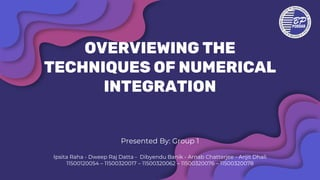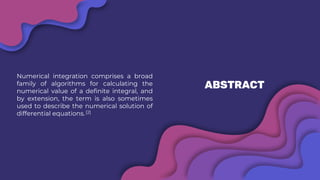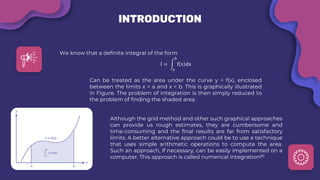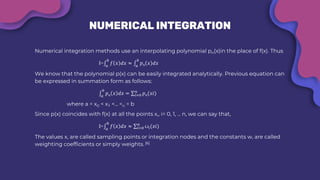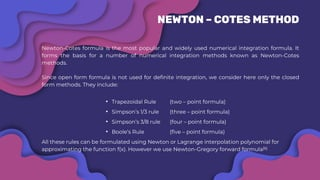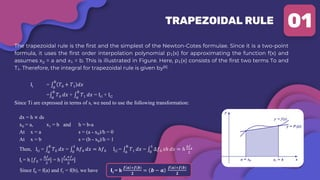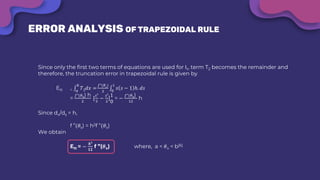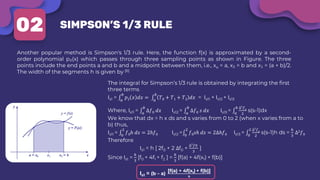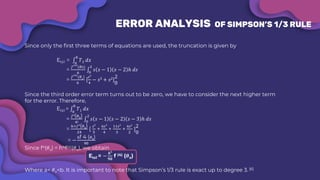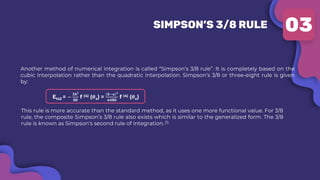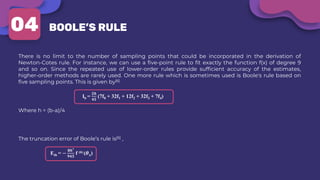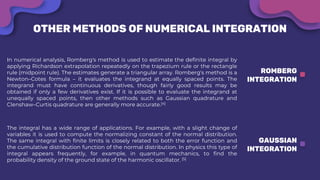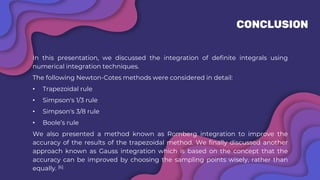1 sur 17
Publicité

### Overviewing the techniques of Numerical Integration.pdf

1. OVERVIEWING THE TECHNIQUES OF NUMERICAL INTEGRATION Presented By: Group 1 Ipsita Raha - Dweep Raj Datta - Dibyendu Banik - Arnab Chatterjee - Arijit Dhali 11500120054 – 11500320017 – 11500320062 – 11500320076 – 11500320078
2. TABLE OF CONTENTS ABSTRACT & KEYWORDS INTRODUCTION MATHEMATICAL ANALYSIS CONCLUSION & REFERENCES 1 2 3 4
3. ABSTRACT Numerical integration comprises a broad family of algorithms for calculating the numerical value of a definite integral, and by extension, the term is also sometimes used to describe the numerical solution of differential equations. 
4. KEYWORDS  Newton-Cotes Method  Integration  Newton-Gregory Method  Lagrange Interpolation
5. INTRODUCTION We know that a definite integral of the form I = න a b f x dx Can be treated as the area under the curve y = f(x), enclosed between the limits x = a and x = b. This is graphically illustrated in Figure. The problem of integration is then simply reduced to the problem of finding the shaded area. Although the grid method and other such graphical approaches can provide us rough estimates, they are cumbersome and time-consuming and the final results are far from satisfactory limits. A better alternative approach could be to use a technique that uses simple arithmetic operations to compute the area. Such an approach, if necessary, can be easily implemented on a computer. This approach is called numerical integration
6. NUMERICAL INTEGRATION Numerical integration methods use an interpolating polynomial pn(x)in the place of f(x). Thus I=‫׬‬𝑎 𝑏 𝑓 𝑥 𝑑𝑥 ≈ ‫׬‬𝑎 𝑏 𝑝𝑛 𝑥 𝑑𝑥 We know that the polynomial p(x) can be easily integrated analytically. Previous equation can be expressed in summation form as follows: ‫׬‬𝑎 𝑏 𝑝𝑛 𝑥 𝑑𝑥 = σ𝑖=0 𝑛 𝑝𝑛(𝑥𝑖) where a = x0 < x₁ <... <n = b Since p(x) coincides with f(x) at all the points x,, i= 0, 1, ... n, we can say that, I=‫׬‬𝑎 𝑏 𝑓 𝑥 𝑑𝑥 ≈ σ𝑖=0 𝑛 ꙍ𝑖(𝑥𝑖) The values x, are called sampling points or integration nodes and the constants w, are called weighting coefficients or simply weights. 
7. NEWTON – COTES METHOD Newton-Cotes formula is the most popular and widely used numerical integration formula. It forms the basis for a number of numerical integration methods known as Newton-Cotes methods. Since open form formula is not used for definite integration, we consider here only the closed form methods. They include: • Trapezoidal Rule (two – point formula) • Simpson’s 1/3 rule (three – point formula) • Simpson’s 3/8 rule (four – point formula) • Boole’s Rule (five – point formula) All these rules can be formulated using Newton or Lagrange interpolation polynomial for approximating the function f(x). However we use Newton-Gregory forward formula
8. TRAPEZOIDAL RULE The trapezoidal rule is the first and the simplest of the Newton-Cotes formulae. Since it is a two-point formula, it uses the first order interpolation polynomial p₁(x) for approximating the function f(x) and assumes x0 = a and x₁ = b. This is illustrated in Figure. Here, p₁(x) consists of the first two terms To and T₁. Therefore, the integral for trapezoidal rule is given by It = ‫׬‬𝑎 𝑏 𝑇0 + 𝑇1 𝑑𝑥 =‫׬‬𝑎 𝑏 𝑇0 𝑑𝑥 + ‫׬‬𝑎 𝑏 𝑇1 𝑑𝑥 = It1 + It2 Since Ti are expressed in terms of s, we need to use the following transformation: dx = h × ds x0 = a, x1 = b and h = b-a At x = a s = (a - x0)/h = 0 At x = b s = (b - x0)/h = 1 Then, It1 = ‫׬‬𝑎 𝑏 𝑇0 𝑑𝑥 = ‫׬‬0 1 ℎ𝑓0 𝑑𝑥 = ℎ𝑓0 It2 = ‫׬‬𝑎 𝑏 𝑇1 𝑑𝑥 = ‫׬‬0 1 ∆𝑓0 𝑠ℎ 𝑑𝑠 = ℎ ∆𝑓0 2 It = h [𝑓0 + ∆𝑓0 2 ] = h [ 𝑓0+𝑓1 2 ] Since f0 = f(a) and f1 = f(b), we have It = h 𝒇 𝒂 +𝒇(𝒃) 𝟐 = 𝒃 − 𝒂 𝒇 𝒂 +𝒇(𝒃) 𝟐 01
9. ERROR ANALYSIS OF TRAPEZOIDAL RULE Since only the first two terms of equations are used for It, term T2 becomes the remainder and therefore, the truncation error in trapezoidal rule is given by Ett = ‫׬‬𝑎 𝑏 𝑇2𝑑𝑥 = 𝑓"(𝜃𝑠) 2 ‫׬‬0 1 𝑠 𝑠 − 1 ℎ. 𝑑𝑠 = 𝑓"(𝜃s) h 2 [ 𝑠3 3 − 𝑠2 2 ] 1 0 = − 𝑓"(𝜃s) 12 h Since dx/ds = h, f ”(𝜃s) = h2f ”(𝜃x) We obtain Ett = − 𝒉𝟑 𝟏𝟐 f ”(𝜽x) where, a < 𝜃x < b
10. SIMPSON’S 1/3 RULE Another popular method is Simpson's 1/3 rule. Here, the function f(x) is approximated by a second- order polynomial p₂(x) which passes through three sampling points as shown in Figure. The three points include the end points a and b and a midpoint between them, i.e., xo = a, x₂ = b and x₁ = (a + b)/2. The width of the segments h is given by  02 The integral for Simpson’s 1/3 rule is obtained by integrating the first three terms Is1 = ‫׬‬𝑎 𝑏 𝑝2 𝑥 𝑑𝑥 = ‫׬‬𝑎 𝑏 𝑇0 + 𝑇1 + 𝑇2 𝑑𝑥 = Is11 + Is12 + Is13 Where, Is11 = ‫׬‬𝑎 𝑏 ∆𝑓0 𝑑𝑥 Is12 = ‫׬‬𝑎 𝑏 ∆𝑓0 𝑠 𝑑𝑥 Is13 = ‫׬‬𝑎 𝑏 ∆2 𝑓0 2 s(s-1)dx We know that dx = h x ds and s varies from 0 to 2 (when x varies from a to b) thus, Is11 = ‫׬‬0 2 𝑓0ℎ 𝑑𝑠 = 2ℎ𝑓0 Is12 =‫׬‬0 2 𝑓0𝑠ℎ 𝑑𝑠 = 2∆ℎ𝑓0 Is13 = ‫׬‬0 2 ∆2 𝑓0 2 s(s-1)h ds = ℎ 3 ∆2𝑓0 Therefore Is1 = h [ 2f0 + 2 ∆f0 + ∆2 𝑓0 3 ] Since Is1 = ℎ 3 [f0 + 4f1 + f2 ] = ℎ 3 [f(a) + 4f(x1) + f(b)] Is1 = (b – a) [f(a) + 4f(x1) + f(b)] 𝟔
11. ERROR ANALYSIS OF SIMPSON’S 1/3 RULE Since only the first three terms of equations are used, the truncation is given by Ets1 = ‫׬‬𝑎 𝑏 𝑇3 𝑑𝑥 = 𝑓′′′(𝜃𝑠) 6 ‫׬‬0 2 𝑠 𝑠 − 1 𝑠 − 2 ℎ 𝑑𝑠 = 𝑓′′′(𝜃𝑠) 6 [ 𝑠4 4 − 𝑠3 + 𝑠2] 2 0 Since the third order error term turns out to be zero, we have to consider the next higher term for the error. Therefore, Ets1 = ‫׬‬𝑎 𝑏 𝑇1 𝑑𝑥 = 𝑓4 (𝜃s) 4! ‫׬‬0 2 𝑠 𝑠 − 1 𝑠 − 2 𝑠 − 3 ℎ 𝑑𝑠 = ℎ×𝑓4 (𝜃s) 24 [ 𝑠5 5 + 6𝑠4 4 + 11𝑠3 3 + 6𝑠2 2 ] 2 0 = − ℎf 4 (𝜃s) 90 Since f4(𝜃s) = h4f(4)(𝜃x), we obtain Ets1 = − 𝒉𝟓 𝟗𝟎 f (4) (𝜽x) Where a< 𝜃x<b. It is important to note that Simpson’s 1/3 rule is exact up to degree 3. 
12. SIMPSON’S 3/8 RULE Another method of numerical integration is called “Simpson’s 3/8 rule”. It is completely based on the cubic interpolation rather than the quadratic interpolation. Simpson’s 3/8 or three-eight rule is given by: Ets2 = − 𝟑𝒉𝟓 𝟖𝟎 f (4) (𝜽x) = 𝒃−𝒂 𝟓 𝟔𝟒𝟖𝟎 f (4) (𝜽x) 03 This rule is more accurate than the standard method, as it uses one more functional value. For 3/8 rule, the composite Simpson’s 3/8 rule also exists which is similar to the generalized form. The 3/8 rule is known as Simpson’s second rule of integration. 
13. BOOLE’S RULE 04 There is no limit to the number of sampling points that could be incorporated in the derivation of Newton-Cotes rule. For instance, we can use a five-point rule to fit exactly the function f(x) of degree 9 and so on. Since the repeated use of lower-order rules provide sufficient accuracy of the estimates, higher-order methods are rarely used. One more rule which is sometimes used is Boole's rule based on five sampling points. This is given by 𝐈b = 𝟐𝒉 𝟒𝟓 (7f0 + 32f1 + 12f2 + 32f3 + 7f4) Where h = (b-a)/4 The truncation error of Boole’s rule is , Etb = − 𝟖𝒉𝟕 𝟗𝟒𝟓 f (6) (𝜽𝒙)
14. OTHER METHODS OF NUMERICAL INTEGRATION GAUSSIAN INTEGRATION The integral has a wide range of applications. For example, with a slight change of variables it is used to compute the normalizing constant of the normal distribution. The same integral with finite limits is closely related to both the error function and the cumulative distribution function of the normal distribution. In physics this type of integral appears frequently, for example, in quantum mechanics, to find the probability density of the ground state of the harmonic oscillator.  ROMBERG INTEGRATION In numerical analysis, Romberg's method is used to estimate the definite integral by applying Richardson extrapolation repeatedly on the trapezium rule or the rectangle rule (midpoint rule). The estimates generate a triangular array. Romberg's method is a Newton–Cotes formula – it evaluates the integrand at equally spaced points. The integrand must have continuous derivatives, though fairly good results may be obtained if only a few derivatives exist. If it is possible to evaluate the integrand at unequally spaced points, then other methods such as Gaussian quadrature and Clenshaw–Curtis quadrature are generally more accurate.
15. CONCLUSION In this presentation, we discussed the integration of definite integrals using numerical integration techniques. The following Newton-Cotes methods were considered in detail: • Trapezoidal rule • Simpson's 1/3 rule • Simpson's 3/8 rule • Boole’s rule We also presented a method known as Romberg integration to improve the accuracy of the results of the trapezoidal method. We finally discussed another approach known as Gauss integration which is based on the concept that the accuracy can be improved by choosing the sampling points wisely, rather than equally. 
16. REFERENCE 1. https://byjus.com/maths/simpsons-rule/ 2. https://math.berkeley.edu/~mgu/MA128ASpring2017/MA128ALectureWeek6.pdf 3. https://en.wikipedia.org/wiki/Numerical_integration 4. https://en.wikipedia.org/wiki/Romberg%27s_method 5. https://en.wikipedia.org/wiki/Gaussian_integral 6. Numerical Methods by E Balagurusamy
17. For your kind attention towards our presentation THANK YOU
Publicité